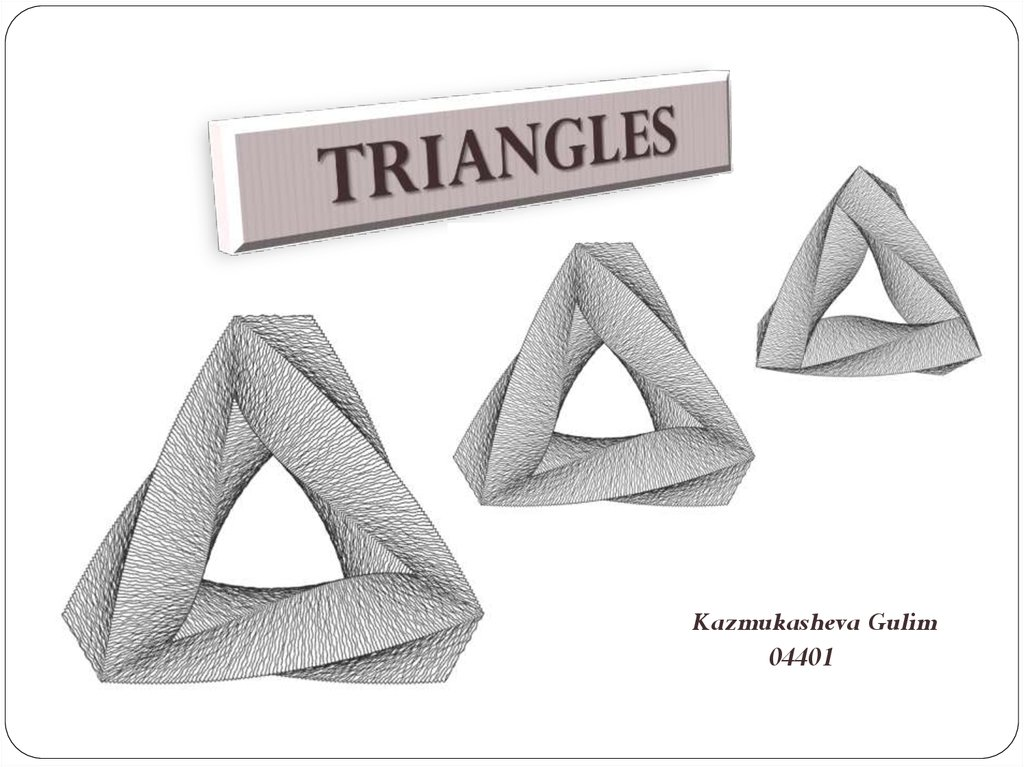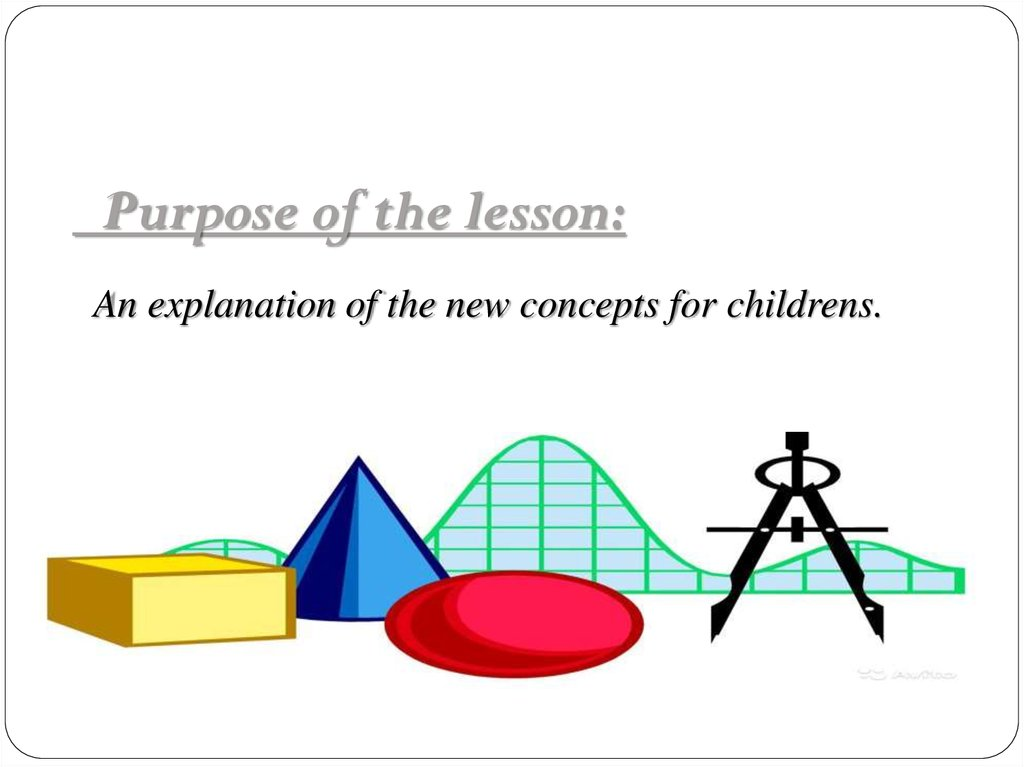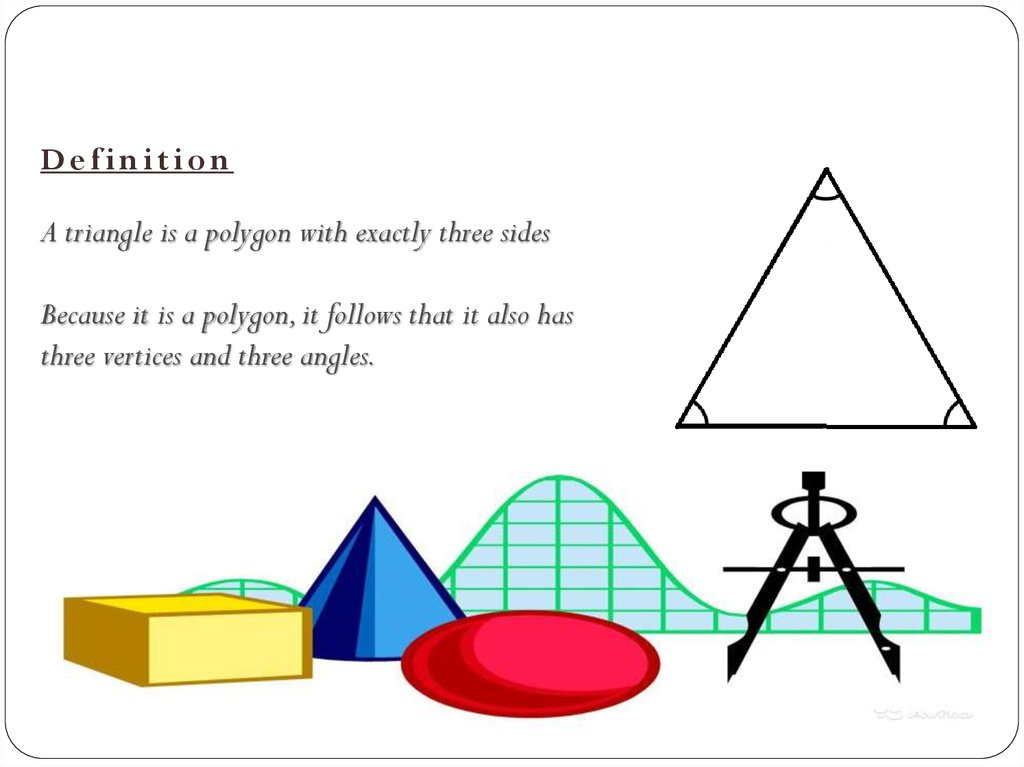Triangle. Definition

1.

Kazmukasheva Gulim
04401

2.

Purpose of the lesson:
An explanation of the new concepts for childrens.

3.

Definition
A triangle is a polygon with exactly three sides
Because it is a polygon, it follows that it also has
three vertices and three angles.

B
h
A
l
m
C
h-height
l- bisector
m- median

5.

Types of Triangle
An isosceles triangle is a triangle in
which two sides are the same length.
An equilateral triangle is
a triangle in which all three sides are the
same length:

6.

A scalene triangle is a triangle in
which all three sides are of different
lengths.
A right-angled triangle is a
triangle in which one of the vertices
is a right angle.

B
A
C